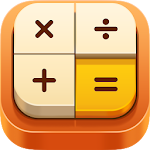ID: jp.co.conduits.calcbas

## The description of Calculator - CASIO style Multi calc with Remainder

It can be used in the same operation as your favorite CASIO calculator.
You can arrange the keys freely, also supports remainder calculation, tax, date/time calculation, history display.
Support for currency exchange conversion, twin display, reading calculator, more convenient.
★ Added the Forex conversion function ★

The calculation method of remainder calculation is adjusted to the CASIO MP-12R.
The basic calculation function emulates the CASIO MS-10VC series.

Real Calculator is an application that allows you to do exactly the same as your favorite calculator.

Main features:
* Works exactly like the CASIO real calculator, making it perfect for accounting, accounting and learning.
* Built in multiple calculators. You can easily change from the menu.
* Currency exchange rates correspond to 168 currencies. The rates are obtained automatically from the server.
* Hours, days and dates can be calculated.
* You can divide the rest with the [÷ R] key.
* Two calculations can be made simultaneously with the double display function.
* You can freely configure the layout of the keys, the screen and the calculation settings, and save it as the calculator configured with a name.
* The calculation history remains, and notes can be added / searched. You can also copy to the clipboard.
* Support for widgets. You can calculate on the home screen without starting the application.
* Three tones of CASIO Tone VL-1 are recorded for the musical performance function.
* The performance of the music corresponds to the operation of AR-7778, a hot topic on YouTube, and VL-80 of the CASIO tradition.
* It has a function to read (speech) the input content and calculation.
* Built in multiple wallpapers. You can also use images on your smartphone.
* You can select between 7 types of button shapes and 3 types of sources for the display of the number (screen).
* You can select from 8 to 14 digits.

Behavior when changing calculator sets:
You can choose between the following actions when changing:
takeover mode: The calculation is maintained even if you change the calculator, so you can use several calculators in a calculation.
(Example) Calculation Calculator → Purchasing Calculator → Practical Calculator
Different mode: Each calculator has its own status, so it can be used as a separate calculator for each set
(Example) Calculator of adjustments of 8% of the tax on consumption and Calculator of adjustments of 10% of the consumption tax

Main calculation functions:
Number of digits: 10 digits / 8 digits / 12 digits / 14 digits Selection formula
Memory: 1 memory (or separate memory for each device)
Screen memory:
Memory / Constant calculation Shows the status of the memory.
GT: Great total function
Currency conversion:
You can convert between up to 4 different currencies. It supports the automatic acquisition rate of 168 currencies.
Sales price / cost / gross margin:
Calculation of the cost / sale price / gross profit rate, which is essential for business, with a touch key
Discount calculation:
You can make% discount and discount calculation. The amount of the discount is also shown.